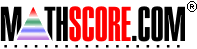Math Practice Online > free > lessons > Florida > 6th grade > Fraction Division

## Fraction Division

For dividing two fractions. You may need pencil and paper.

 Sample Problems for Fraction Division Lesson for Fraction Division

### This topic aligns to the following state standards

Grade 5: Num 4. Explains and demonstrates the inverse nature of multiplication and division, with particular attention to multiplication by a fraction (for example, multiplying by 3/4 yields the same result as dividing by 4).
Grade 6: Num 1. Knows the effects of the four basic operations on whole numbers, fractions, mixed numbers, and decimals.
Grade 7: Num 1. Knows the effects of the four basic operations on whole numbers, fractions, mixed numbers, and decimals.
Grade 8: Num 1. Knows the effects of the four basic operations on whole numbers, fractions, mixed numbers, decimals, and integers.

Copyright Accurate Learning Systems Corporation 2008.